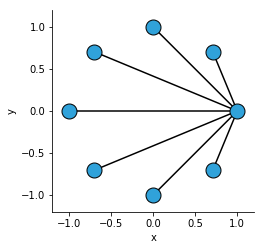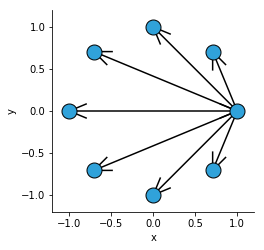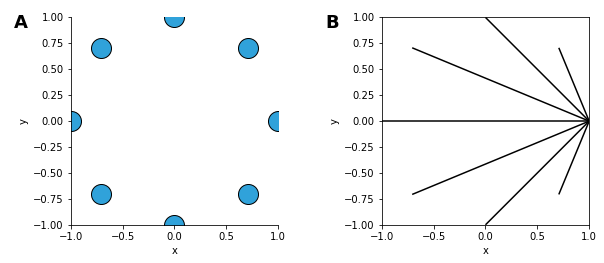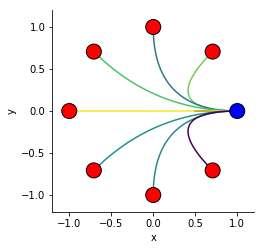# Graph¶

Title
Graph Element
Dependencies
Matplotlib
Backends
Matplotlib
Bokeh
In :
import numpy as np
import holoviews as hv
from holoviews import opts

hv.extension('matplotlib')The Graph element provides an easy way to represent and visualize network graphs. It differs from other elements in HoloViews in that it consists of multiple sub-elements. The data of the Graph element itself are the abstract edges between the nodes. By default the element will automatically compute concrete x and y positions for the nodes and represent them using a Nodes element, which is stored on the Graph. The abstract edges and concrete node positions are sufficient to render the Graph by drawing straight-line edges between the nodes. In order to supply explicit edge paths we can also declare EdgePaths, providing explicit coordinates for each edge to follow.

To summarize a Graph consists of three different components:

• The Graph itself holds the abstract edges stored as a table of node indices.
• The Nodes hold the concrete x and y positions of each node along with a node index. The Nodes may also define any number of value dimensions, which can be revealed when hovering over the nodes or to color the nodes by.
• The EdgePaths can optionally be supplied to declare explicit node paths.

This reference document describes only basic functionality, for a more detailed summary on how to work with network graphs in HoloViews see the User Guide.

#### A simple Graph¶

Let's start by declaring a very simple graph connecting one node to all others. If we simply supply the abstract connectivity of the Graph, it will automatically compute a layout for the nodes using the layout_nodes operation, which defaults to a circular layout:

In :
# Declare abstract edges
N = 8
node_indices = np.arange(N)
source = np.zeros(N)
target = node_indices

padding = dict(x=(-1.2, 1.2), y=(-1.2, 1.2))

simple_graph = hv.Graph(((source, target),))

Out:#### Directed graphs¶

The Graph element also allows indicating the directionality of graph edges using arrows. To enable the arrows set directed=True and to optionally control the arrowhead_length provide a length as a fraction of the total graph extent:

In :
simple_graph.opts(directed=True, arrowhead_length=0.08)

Out:#### Accessing the nodes and edges¶

We can easily access the Nodes and EdgePaths on the Graph element using the corresponding properties:

In :
simple_graph.nodes + simple_graph.edgepaths

Out:Next we will extend this example by supplying explicit edges, node information and edge weights. By constructing the Nodes explicitly we can declare an additional value dimensions, which are revealed when hovering and/or can be mapped to the color by specifying the color. We can also associate additional information with each edge by supplying a value dimension to the Graph itself, which we can map to a color using the edge_color.

In :
# Node info
np.random.seed(7)
x, y = simple_graph.nodes.array([0, 1]).T
node_labels = ['Output']+['Input']*(N-1)
edge_weights = np.random.rand(8)

# Compute edge paths
def bezier(start, end, control, steps=np.linspace(0, 1, 100)):
return (1-steps)**2*start + 2*(1-steps)*steps*control+steps**2*end

paths = []
for node_index in node_indices:
ex, ey = x[node_index], y[node_index]
paths.append(np.column_stack([bezier(x, ex, 0), bezier(y, ey, 0)]))

# Declare Graph
nodes = hv.Nodes((x, y, node_indices, node_labels), vdims='Type')
graph = hv.Graph(((source, target, edge_weights), nodes, paths), vdims='Weight')

graph.opts(
opts.Graph(cmap=['blue', 'red'], edge_cmap='viridis', edge_color='Weight', node_color='Type', padding=0.1))

Out:For full documentation and the available style and plot options, use hv.help(hv.Graph).